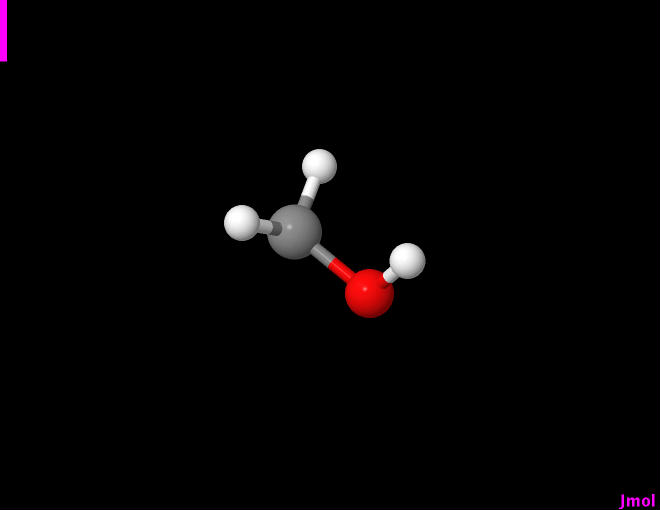# CH2OH

Click on the   icon info to get details.

Species data
 Common Formula CH2OH Stoichiometric Formula CH2OH Name hydroxymethyl radical Mass 31.01839 a.m.u Charge 0 CAS 2597-43-5 Inchi InChI=1S/CH3O/c1-2/h2H,1H2 InchiKey CBOIHMRHGLHBPB-UHFFFAOYSA-N State Ground StateISM Abundance
log10 Abundance Reference Source Name Source Type Link
Polarizability
No data
Dipole moment
No data
Enthalpy of formation
 T (K): 0 Value (kJ.mol-1) : -10.7 ±0.44 Method: Reviews and Evaluations Origin: Other database Reference: T (K): 298 Value (kJ.mol-1) : -17 ±0.44 Method: Reviews and Evaluations Origin: Other database Reference:
Desorption energy
 Emean (K): 4400 ±1320 E min (K): 0 E max (K): 0 Pre-exponential factor (s-1): 0.00E+0 Method: Calculations Origin: Bibliography Reference: Wakelam, V. et al. ;2017;ArXiv e-prints;, Type of surface: H2O Description: To estimate the unknown binding energies (for most of the radicals for example), we have developed a model founded on the stabilization energy of the complex between the various species and one water molecule. Then, we assume that the binding energy of the species with ASW is proportional to the energy of interaction between this species and one water molecule. To determine the proportionality coefficients, we fit the dependency of the experimental binding energies versus the calculated energies of the complexes for 16 stable molecules. Uncertainties in ED is estimated to be 30%. The preexponential factor is to be computed using the Hasegawa et al. (1992) approximation. Evaluation: Emean (K): 5084 E min (K): 0 E max (K): 0 Pre-exponential factor (s-1): 0.00E+0 Method: Estimation Origin: Other database Reference: Type of surface: H2O Description: This binding energy was listed in the original OSU gas-grain code from Eric Herbst group in 2006. Energy of CH3OH - H. The pre-exponential factor is not given. It can be computed using the formula given in Hasegawa et al. (1992). Evaluation:
Diffusion energy
No data